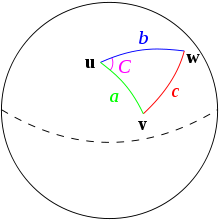# Spherical law of cosines

In spherical trigonometry, the law of cosines (also called the cosine rule for sides) is a theorem relating the sides and angles of spherical triangles, analogous to the ordinary law of cosines from plane trigonometry.Spherical triangle solved by the law of cosines.

Given a unit sphere, a "spherical triangle" on the surface of the sphere is defined by the great circles connecting three points u, v, and w on the sphere (shown at right). If the lengths of these three sides are a (from u to v), b (from u to w), and c (from v to w), and the angle of the corner opposite c is C, then the (first) spherical law of cosines states:Since this is a unit sphere, the lengths a, b, and c are simply equal to the angles (in radians) subtended by those sides from the center of the sphere. (For a non-unit sphere, the lengths are the subtended angles times the radius, and the formula still holds if a, b and c are reinterpreted as the subtended angles). As a special case, for C = π/2, then cos C = 0, and one obtains the spherical analogue of the Pythagorean theorem:If the law of cosines is used to solve for c, the necessity of inverting the cosine magnifies rounding errors when c is small. In this case, the alternative formulation of the law of haversines is preferable.

A variation on the law of cosines, the second spherical law of cosines, (also called the cosine rule for angles) states:where A and B are the angles of the corners opposite to sides a and b, respectively. It can be obtained from consideration of a spherical triangle dual to the given one.

## Proofs

### First proof

A proof of the law of cosines can be constructed as follows. Let u, v, and w denote the unit vectors from the center of the sphere to those corners of the triangle. Then, the lengths (angles) of the sides are given by the dot products:To get the angle C, we need the tangent vectors ta and tb at u along the directions of sides a and b, respectively. For example, the tangent vector ta is the unit vector perpendicular to u in the u-v plane, whose direction is given by the component of v perpendicular to u. This means:where for the denominator we have used the Pythagorean identity sin2 a = 1 − cos2 a and where || || denotes the length of the vector in the denominator. Similarly,Then, the angle C is given by:from which the law of cosines immediately follows.

### Second proof

To the diagram above, add a plane tangent to the sphere at u, and extend radii from the center of the sphere O through v and through w to meet the plane at points y and z. We then have two plane triangles with a side in common: the triangle containing u, y and z and the one containing O, y and z. Sides of the first triangle are tan a and tan b, with angle C between them; sides of the second triangle are sec a and sec b, with angle c between them. By the law of cosines for plane triangles (and remembering that sec2 of any angle is tan2 + 1),SoMultiply both sides by cos a cos b and rearrange.

### Third proof

The angles and distances do not change if the sphere is rotated, so we can rotate the sphere so thatis at the north pole andis somewhere on the prime meridian (longitude of 0). With this rotation, the spherical coordinates forareand the spherical coordinates forare. The Cartesian coordinates forareand the Cartesian coordinates forare. The value ofis the dot product of the two Cartesian vectors, which is.

## Rearrangements

The first and second spherical laws of cosines can be rearranged to put the sides and angles on opposite sides of the equations:## Planar limit: small angles

For small spherical triangles, i.e. for small a, b, and c, the spherical law of cosines is approximately the same as the ordinary planar law of cosines,To prove this, we will use the small-angle approximation obtained from the Maclaurin series for the cosine and sine functions:Substituting these expressions into the spherical law of cosines nets:or after simplifying:Remembering the properties of big O notation, we can discard summands where the lowest exponent for a or b is greater than 1, so finally, the error in this approximation is: